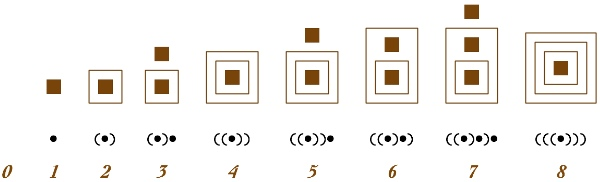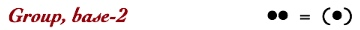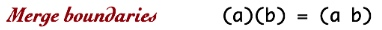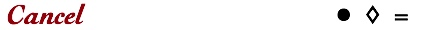# Binary CalculatorThe Iconic Arithmetic Calculator set to binary mode shows computation using base-2 container numbers. The Image shows three binary numbers that have been added together, two are negative numbers. Positive units are indicated by full squares, , while negative units are indicated by empty diamonds, . Iconic arithmetic uses the presence of a unit in a container to indicate one, while the absence of a unit indicates zero. Binary numbers are particularly convenient for showing how iconic arithmetic works. When two units share the same space, they are grouped together as a single unit in a new container that doubles its contents. Grouping allows us to efficiently refer to large unit ensembles. Other than grouping size, the rules of iconic arithmetic are the same in all bases. The addition shown in the image (435+(–45)+(–165) in decimal notation) is animated below as an introduction to the Binary Calculator. For context, the entire sequence of entering these three numbers into the calculator is included for this first example. The rest of the animations then show the calculator display window only. These examples illustrate the details of how iconic binary addition works. The transformations shown in each video are also expressed in parens notation on the Parens Notation page. The Container Numbers page provides guidance about how to read binary container numbers.

The binary iconic calculator: 435+(–45)+(–165)=225

The iconic binary numbers from 0 to 8 are shown below in two notations, the two dimensional notation used in the animations, and the one dimensional parens notation.Positive and negative numbers are added by putting them into the same container. Three rules (Group, Merge, Cancel) convert the result of addition into a standard factored form. Below, these rules are defined by iconic equations, and then illustrated by animations.

Grouping joins two units together in one container, exchanging multiplicity of units for multiplication by bounding.Group two units by adding 1 to 1

Merging combines boundaries at the same level of nesting. Any number of boundaries can merge at the same time. The animation shows a pair of boundaries, each containing a single unit, merging.Merge boundaries by adding 2 to 2

Canceling deletes two units of opposite polarity. A positive and a negative unit join together to annihilate one another. A void space remains. Void in iconic notation means zero in symbolic notation.Cancel opposites by adding 1 to –1

This video shows four units being standardized by the Group and Merge rules. Units Group in pairs, the resulting boundaries Merge, and the innermost two units that result from this Merge operation Group again.

When multiple boundaries are in the same space, they Merge concurrently. Here eight copies of 2 are merged, with all boundary merges occurring at the same time. The resulting single boundary contains eight single units, which Group by twos, again all at the same time. This results in four boundaries. The oscillation of boundary merging and unit grouping continues, each time reducing the number of units by a factor of two. This example illustrates how computation includes both in parallel and in sequential steps. Parallel actions can generate new structure which in turn can trigger another sequential round of parallel actions. Each time parallel actions occur, the conditions for a new sequential step might be generated. In this example, eighteen instances of standardization occur, eleven are Merges and seven are Groupings. All of these occur in five sequential steps.

The Merge operation is not restricted to outer boundaries. In contrast to the animations shown in Container Numbers, the Iconic Calculator merges all nested levels concurrently. Here 8+8 shows three levels of nesting merging at the same time. Standardization of the sum requires three Merges and one Group, all take place in two steps.

Both Container Numbers and Network Numbers show the binary addition 111+101=1100, which is 7+5=12 in decimal. The animation below differs from the Container Numbers animation by including more parallelism. In all cases, the binary addition 7+5 requires three Group actions and four Merge actions if we count actions as single steps. The animation below, however, shows all depths merging concurrently. The seven operations require five sequential steps.

The interplay of concurrency and sequence cannot easily be expressed in linear notation (be it conventional or parens). The dynamics of parallelism and sequence are best displayed and comprehended through spatial animation. A central point is that writing ideas as strings of characters on lines imposes structural limitations on those ideas. Textual notation limits conceptual understanding. It is not possible to separate notation from concept. This happens in two ways. Notation can introduce accidental structure not intended to be part of the concept being expressed. And notation can restrict concept by being unable to convey aspects of the concept being expressed.

We are now in position to explore the various dynamic sequences that occur during binary addition. Although we will not cover the details here, characterization of these sequences can contribute to the analysis of the behavior of computer circuitry. Some circuits require excessive energy, for example, and can run down the battery of a portable device more rapidly. Often they can be transformed into more efficient circuitry.

When the speedometer rolls over from 9999 to 10000, every digit changes. In binary, we can see the propagation of Group and Merge as a number goes from one less than a power of two to that power. Here is 31+1.

It is possible to add binary numbers that fit together without triggering any Merge operations. Here is 545+154+324

545+154+324=1023

In this example, most merged spaces contain two units, so each enacts a Group operation. Then no further grouping occurs.

51+348+110=509

Here is the sum of digits from 1 to 8.

Adding the digits from 1 to 8

Here’s an addition with lots of parallel activity.

A final example of binary addition that emphasizes parallelism.

1618+261+4401=6280

## Exploring Binary Subtraction

Negative binary numbers add another dynamic to the display of binary arithmetic. We begin with subtraction 1 from 2, expressed as the addition 2+(–1).

Subtracting 1 from 2

The behavior of binary iconic arithmetic does not change when the signs of numbers are changed. Here is 1–2, expressed as 1+(–2).

Subtracting 2 from 1

The Cancel rule works only when a positive and a negative unit share the same container. The animation of 2–1 above shows that a unit must first be Ungrouped so that opposite units are together in the outermost container. Then the Cancel rule applies to delete units in pairs. Here is a more extreme example that shows the necessity of Unmerging and Ungrouping units from deeper nested levels.

Subtracting 1 from 32

Canceling can occur in parallel at multiple levels of nesting.

215–87=128

Canceling can also occur at the deepest levels of nesting.

235–225=10

Localized Unmerge and Ungroup propagates only until an opposite unit is reached. Here the example also shows that the standardization process is insensitive to which number is larger, positive or negative.

42–213=–171

## Mixed Polarity Numbers

There are two aspects of iconic numbers that set them apart from conventional place-value numbers. Both have already been exhibited. The first aspect is that as many positive and negative numbers as desired can be added at the same time. The second is that once positive and negative numbers have been added together, but prior to standardization, an iconic number exists in a mixed state, with some levels of containment being positive and some being negative. It is depth-value notation that permits each nesting level (that is, each order of magnitude) to be relatively independent of the others. Each level is a separate addition, so that each level may include several units and each level can have a positive or a negative net value. Standardization acts to express each number in a single polarity, either positive or negative. To cancel all of one polarity, it is necessary to propagate the dominant polarity upward until all units of the other polarity have been eliminated. This does not, however, stop parallel operations from occurring at each separate level regardless of the content of the level. In this example, eight small numbers are added together, four positive and four negative. While five separate cancellations are occurring, levels with a net of two or more units of the same polarity proceed in parallel with grouping. In this example, two negative units group and merge into a deeper positive level which triggers another cancellation, without the concept of borrowing.

9–4+5–3–8–7+1+9=2

In this similar example, there are insufficient negative units to Group and Merge into a deeper level, so the positive unit at the deeper level must Unmerge and propagate to the shallower level containing the negative unit.

9–4+5–3–8–5+1+9=4

In this example, the negative levels engage is substantial Grouping prior to being Cancelled. Each Grouping action serves to simplify the remaining form, so that Grouping prior to Canceling is not wasted effort. Without Grouping of different polarities at different levels, an excessive amount of “borrowing” would need to occur.

9–1+9–7–6–6–6+9=1

Here is an example for which positive and negative units cancel exactly to zero. However, there is a great deal of Grouping and Merging activity serving to organize the levels prior to the final cancellation.

200–20–90–90=0

The prior examples are exceptions in that they exhibit special structures. The more common case is a mixture of all the actions we have already seen. This example combines many of the features that were isolated in previous examples. That all actions can happen in parallel indicates that each transformation is independent of the others.

234–45+329–171–164+10=193

Finally an example incorporating relatively large numbers.

211027–49037+48321–209808+90787=91290

Putting the five numbers into the same container (the frame of the image) achieves their addition. Boundary Merging then combines the separate containers into a single container. After merging, each nested level contains from zero to five units, depending upon the contribution to that level by each of the original numbers. Since there are three positive numbers and two negative numbers, each level will contain at most three positive units and two negative units. The next standardization step Cancels pairs of opposite polarity, so that each level has no remaining mixed polarity. At the same time, levels with two remaining units, necessarily of the same polarity, Group and Merge to the next deeper level. Prior to this Merge, there are only four possibilities at each level: one positive unit, one negative unit, no units, or a bounded unit in the process of Merging and thus leaving that particular level. The remaining dynamics of the standardization process is determined by the interleaving of positive and negative levels. The polarity of the deepest level determines the polarity of the sum. Units of that polarity will Unmerge upward to Cancel units of the opposite polarity at shallower levels. The parallel and sequential processes of this example include the entire dynamic range of binary addition.

Each of the video transformation sequences are shown in parens notation on the Parens Notation page.